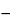Ergocalciferol Oral Solution
DEFINITION
Ergocalciferol Oral Solution is a solution of Ergocalciferol in an edible vegetable oil, in Polysorbate 80, or in Propylene Glycol. It contains NLT 100.0% and NMT 120.0% of the labeled amount of vitamin D as ergocalciferol (C28H44O).
ASSAY
•  Procedure
Mobile phase:  Chloroform containing alcohol as a preservative
Standard stock solution:  50 µg/mL of USP Ergocalciferol RS in chloroform. [Note—Prepare this solution fresh daily. ]
Standard solution A:  5 µg/mL of USP Ergocalciferol RS from the Standard stock solution in chloroform. [Note—Store this Standard solution A at a temperature not above 0. ]
Standard solution B:  Transfer 5.0 mL of the Standard stock solution into a round-bottomed flask fitted with a reflux condenser. Displace the air with nitrogen, and reflux for 1 h in a water bath under a nitrogen atmosphere to obtain a solution containing ergocalciferol and pre-ergocalciferol. Cool, transfer, with the aid of several portions of chloroform, to a 50-mL volumetric flask, dilute with chloroform to volume, and mix.
Sample solution:  Equivalent to 5 µg/mL of ergocalciferol from an accurately measured volume of Oral Solution in chloroform
Chromatographic system
Mode:  LC
Detector:  UV 254 nm
Column:  4.6-mm × 25-cm; 5-µm packing L3
Flow rate:  1 mL/min
Injection size:  10–20 µL
System suitability
Sample:  Standard solution B
[Note—The relative retention times for pre-ergocalciferol and for ergocalciferol are 0.8 and 1.0, respectively. ]
Suitability requirements
Resolution:  NLT 1.0 between the pre-ergocalciferol peak and the ergocalciferol peak
Relative standard deviation:  NMT 2.0%
Analysis
Samples:  Standard solution A, Standard solution B, and Sample solution
Ergocalciferol response factor
Calculate the Ergocalciferol response factor, FD:
FD = CS/rS
 CS = = concentration of USP Ergocalciferol RS in the Standard solution A (µg/mL) rS = = peak response of ergocalciferol from Standard solution A
Pre-ergocalciferol response factor
Calculate the concentration, C¢S, in µg/mL, of ergocalciferol in Standard solution B:
C¢S = FD × r¢S
 FD = = Ergocalciferol response factor r¢S = = peak area of ergocalciferol from Standard solution B
Calculate the concentration, C¢pre, in µg/mL, of pre-ergocalciferol:
C¢pre = CSC¢S
 CS = = concentration of USP Ergocalciferol RS in the Standard solution A (µg/mL) C¢S = = concentration of ergocalciferol in the Standard solution B (µg/mL)
Calculate the Pre-ergocalciferol response factor, Fpre:
Fpre = C¢pre/rp
 C¢pre = = concentration of pre-ergocalciferol (µg/mL) rp = = peak response of pre-ergocalciferol from Standard solution B
Vitamin D content
Calculate the percentage of the labeled amount of vitamin D as ergocalciferol (C28H44O) in the portion of Oral Solution taken:
Result = {[(FD × rC) + (Fpre × rpre)]/CU} × 100
 FD = = ergocalciferol response factor rC = = peak area of ergocalciferol from the Sample solution Fpre = = response factor for pre-ergocalciferol rpre = = peak area of pre-ergocalciferol from the Sample solution CU = = nominal concentration of ergocalciferol in the Sample solution (µg/mL)
Acceptance criteria:  100.0%–120.0%